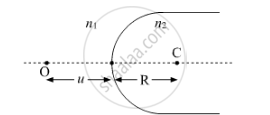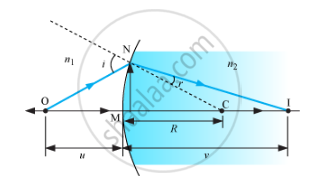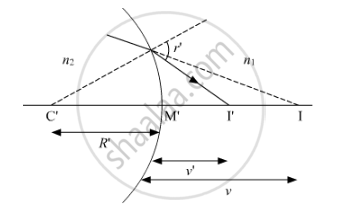Share

# A Point Object 'O' is Kept in a Medium of Refractive Index N1 in Front of a Convex Spherical Surface of Radius of Curvature R Which Separates the Second Medium of Refractive Index N2 from the First One - CBSE (Science) Class 12 - Physics

#### Question

A point object 'O' is kept in a medium of refractive index n1 in front of a convex spherical surface of radius of curvature R which separates the second medium of refractive index n2 from the first one, as shown in the figure

Draw the ray diagram showing the image formation and deduce the relationship between the object distance and the image distance in terms of n1, n2 and R.When the image formed above acts as a virtual object for a concave spherical surface separatig the medium n2 from n1 (n2 > n1), draw this ray diagram and write the similar (similar to (a)) relation. Hence obtain the expression for the lens maker's formula.

#### SolutionLet a spherical surface separate a rarer medium of refractive index n1 from second medium of refractive index n2. Let C be the centre of curvature and R = MC be the radius of the surface.

Consider a point object O lying on the principle axis of the surface. Let a ray starting from O incident normally on the surface along OM and pass straight. Let another ray of light incident on NM along ON and refract along NI.

From M, draw MN perpendicular to OI

The above figure shows the geometry of formation of image I of an object O and the principal axis of a spherical surface with centre of curvature C and radius of curvature R.

Let us make the following assumptions:

(i) The aperture of the surface is small as compared to the other distance involved.

(ii) NM will be taken as nearly equal to the length of the perpendicular from the point N on the principal axis.

tan/_NOM=(MN)/(OM)

tan/_NCM=(MN)/(MC)

tan/_NIM=(MN)/(MI)

For ΔNOC, i is the exterior angle

i = ∠NOM + ∠NCM

For small angles,

i=(MN)/(OM)+(MN)/(MC)

Similarly, r = ∠NCM − ∠NIM

=>r=(MN)/(MC)-(MN)/(MI)

By Snell’s law,

n1sini = n2sinr

For small angles,

n1i= n2 r

Substituting the values of i and r from (i) and (ii), we obtain

n_1((MN)/(OM)+(MN)/(MC))=n_2((MN)/(MC)-(MN)/(MI))

or

n_1/(OM)+n_2/(MI)=(n_2-n_1)/(MC)

Applying new Cartesian sign conventions, we get

OM = −u, MI = +v, MC = +R

Substituting these in (iii), we obtain

n_2/v-n_1/u=(n_2-n_1)/R " ...(iv)"Now, the image I' acts as virtual object for the second surface that will form real image at I. As refraction takes place from denser to rarer medium

:.(-n2)/v+(n1)/(v')=(n2-n1)/(-R')

Adding (iv) and (v), we get

(n1)/(v')-(n1)/u=(n_2-n_1)[1/R-1/(R')]

1/f=(n_21-1)[1/R-1/(R')] "" "[.:n_21=(n2)/(n1),1/f=1/(v')-1/u]

Is there an error in this question or solution?

#### Video TutorialsVIEW ALL 

Solution A Point Object 'O' is Kept in a Medium of Refractive Index N1 in Front of a Convex Spherical Surface of Radius of Curvature R Which Separates the Second Medium of Refractive Index N2 from the First One Concept: Lensmaker's Formula.
S